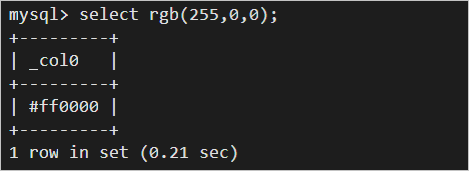This topic describes the syntax of color functions. This topic also provides examples on how to use color functions.

Notice
• If you want to use strings in analytic statements, you must enclose the strings in single quotation marks (''). Strings that are not enclosed or are enclosed in double quotation marks ("") indicate field names or column names. For example, 'status' indicates the status string, and status or "status" indicates the status log field.
• When you use color functions in the Log Service console, the display of query and analysis results is compromised. To avoid this issue, we recommend that you view the query and analysis results on your server.
• Display of query and analysis results in the console• Display of query and analysis results on a server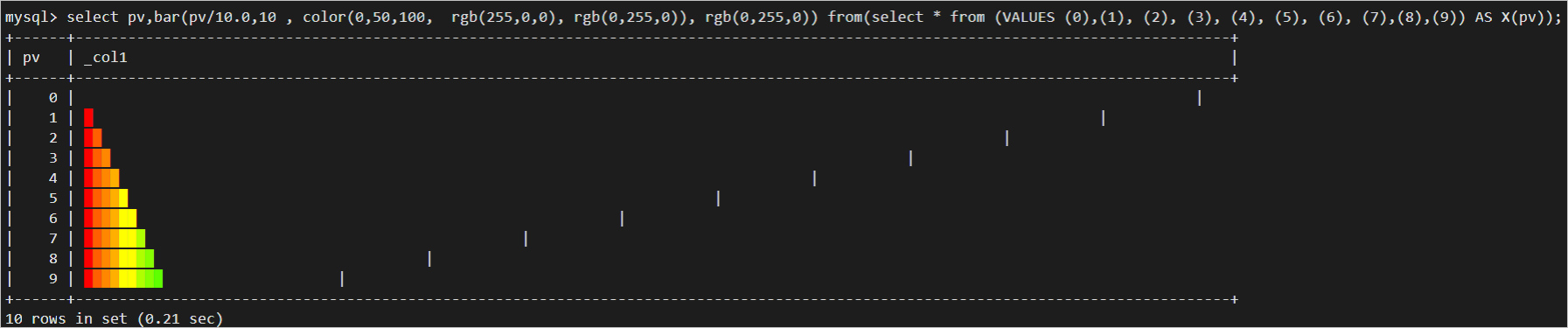Function Syntax Description
bar function bar(x,width) Returns a part of an ANSI bar chart. You can configure the width parameter to specify the width of the ANSI bar chart. However, you cannot configure the high_color or low_color parameter to specify the colors for the chart. The default values of the high_color and low_color parameters are used. The default value of the low_color parameter is red, and the default value of the high_color parameter is green. In addition, you can configure x to specify the length of the part that is returned by the function.
bar(x,width,low_color,high_color) Returns a part of an ANSI bar chart. You can configure the width parameter to specify the width of the ANSI bar chart. You can also configure the high_color and low_color parameters to specify custom colors for the chart. In addition, you can configure x to specify the length of the part that is returned by the function.
color function color(string) Converts a color string to a color type.
color(x,low,high,low_color,high_color) Returns a color between high_color and low_color based on the portions of high_color and low_color. The portions are determined by the proportion of x between high and low.
color(y,low_color, high_color) Returns a color between high_color and low_color based on the portions of high_color and low_color. The portions are determined by y.
render function render(boolean expression) Returns results by using color rendering. If the Boolean expression evaluates to true, the function returns a green tick. If the Boolean expression evaluates to false, the function returns a red cross.
render(x,color) Returns results by using custom color rendering.
rgb function rgb(red,green,blue) Returns a color value based on an RGB value.

## bar function

The bar function returns an ANSI bar chart.

### Syntax

• The following function returns a part of an ANSI bar chart. You can configure the width parameter to specify the width of the ANSI bar chart. However, you cannot configure the high_color or low_color parameter to specify the colors for the chart. The default values of the high_color and low_color parameters are used. The default value of the low_color parameter is red, and the default value of the high_color parameter is green. In addition, you can configure x to specify the length of the part that is returned by the function.
``bar(x,width)``
• The following function returns a part of an ANSI bar chart. You can configure the width parameter to specify the width of the ANSI bar chart. You can also configure the high_color and low_color parameters to specify custom colors for the chart. In addition, you can configure x to specify the length of the part that is returned by the function.
``bar(x,width,low_color,high_color)``

### Parameters

Parameter Description
x The proportion of the part that is returned by the function to the ANSI bar chart. The value of this parameter is of the double type. Valid values: [0,1].
width The width of the ANSI bar chart.
low_color The RGB value of the start color.
high_color The RGB value of the end color.

### Return value type

The varchar type.

### Examples

• Example 1: Obtain a part of an ANSI bar chart based on the proportion of page views (PVs) within a specified hour to the total PVs.
• Query statement
``````* |
SELECT
Method,
bar(pv/m,100)
FROM(
SELECT
*,
max(pv) over() AS m
FROM(
SELECT
Method,
count(1) AS pv
FROM         internal-operation_log
WHERE
__date__ > '2021-09-10 00:00:00'
AND __date__ < '2021-09-10 01:00:00'
GROUP BY
Method
)
)``````
• Query and analysis results (console)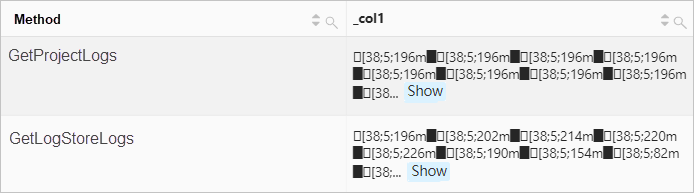• Query and analysis results (server)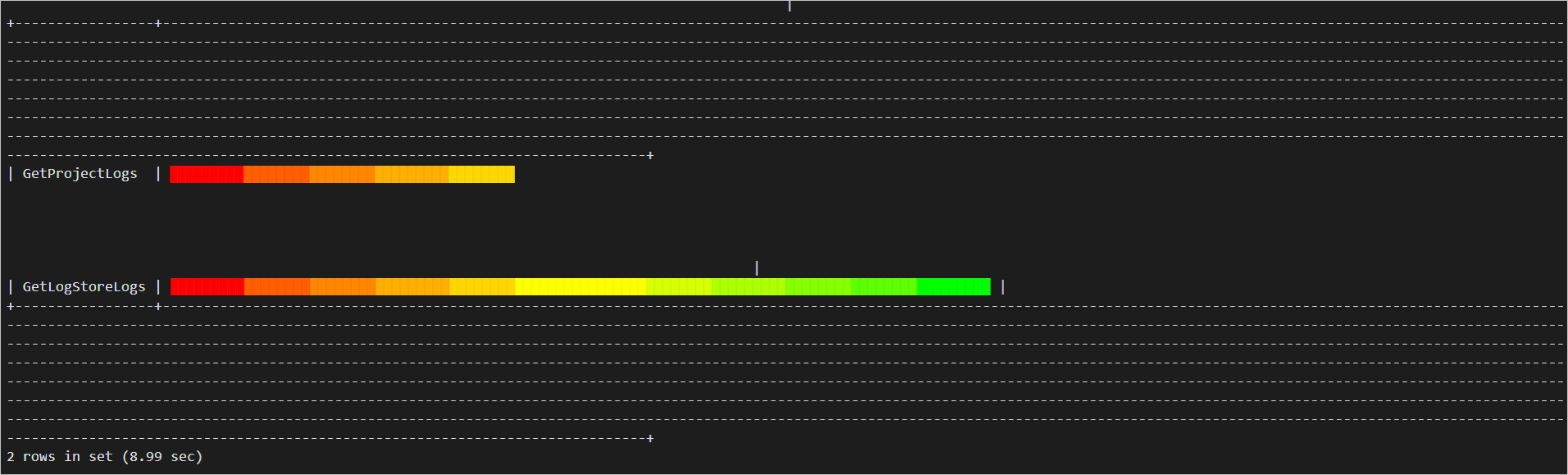• Example 2: Obtain an ANSI bar chart that is displayed in red and white and has a width of 50.
• Query statement
``* | SELECT bar(1,50,rgb(255,255,255),rgb(255,0,0))``
• Query and analysis results (console)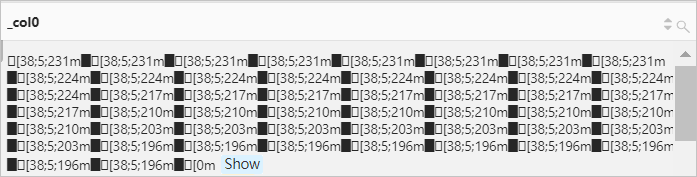• Query and analysis results (server)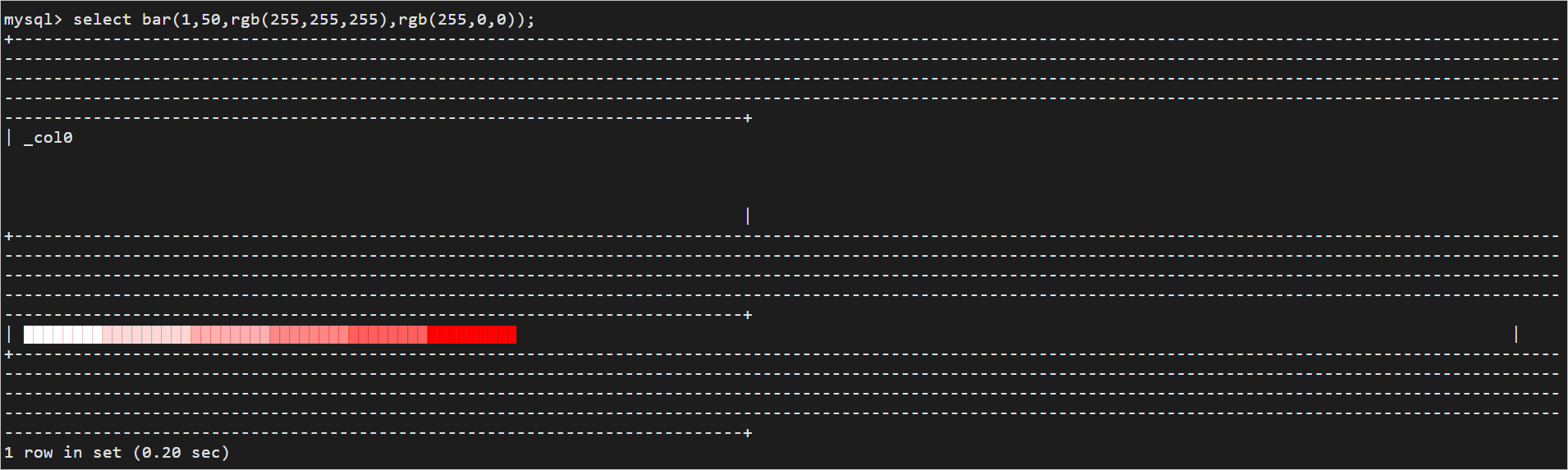## color function

The color function returns the color that corresponds to a value.

### Syntax

• The following function converts a color string to a color type:
``color(string)``
• The following function returns a color between high_color and low_color based on the portions of high_color and low_color. The portions are determined by the proportion of x between high and low.
``color(x,low,high,low_color,high_color)``
• The following function returns a color between high_color and low_color based on the portions of high_color and low_color. The portions are determined by y.
``color(y,low_color,high_color)``

### Parameters

Parameter Description
x The value of this parameter is of the double type.
y The value of this parameter is of the double type. Valid values: [0,1].
low The minimum value. The value of this parameter is of the double type.
high The maximum value. The value of this parameter is of the double type.
low_color The RGB value of the start color.
high_color The RGB value of the end color.
string The string. Valid values: black, red, green, yellow, blue, magenta, cyan, and white. The value can also be an RGB value in the Cascading Style Sheet (CSS) format. Example: #000.

The color type.

### Examples

• Example 1: Convert a color string to a color type.
• Query statement
``* | SELECT color('#000')``
• Query and analysis results (console)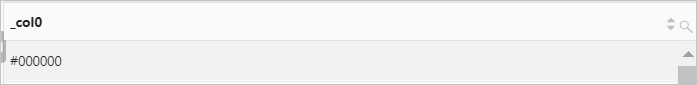• Query and analysis results (server)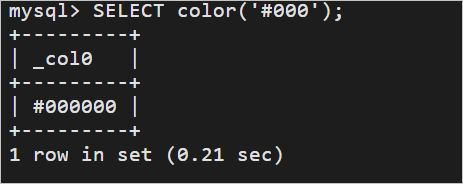• Example 2: Obtain a part of an ANSI bar chart. The remainder of the request_length field value is calculated. Then, the color function returns a color that corresponds to the remainder, and the bar function returns a part of an ANSI bar chart based on the color.
• Query statement
``*|SELECT x,bar(10,10, color(x, 0,10, rgb(255,0,0), rgb(0,255,0)), rgb(0,255,0)) FROM(SELECT  *FROM (SELECT  request_length%10 x FROM  log))``
• Query and analysis results (console)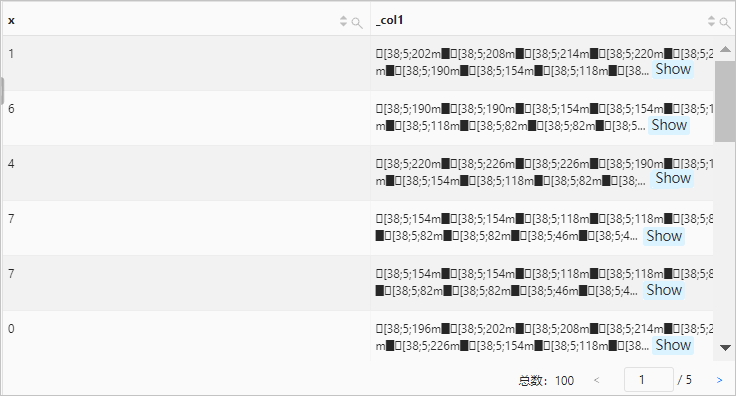• Query and analysis results (server)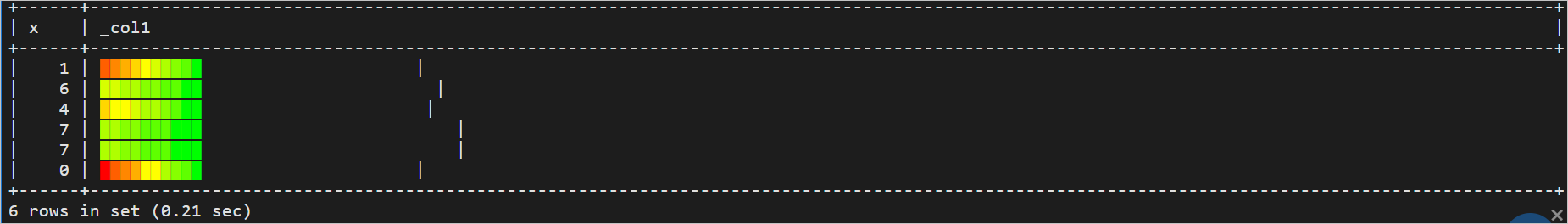• Example 3: Obtain a part of an ANSI bar chart. The color function returns a color, and the bar function returns a part of an ANSI bar chart based on the color.
• Query statement
``*|SELECT bar(10,10, color(0.3, rgb(255,255,255), rgb(255,0,0)), rgb(0,255,0))``
• Query and analysis results (console)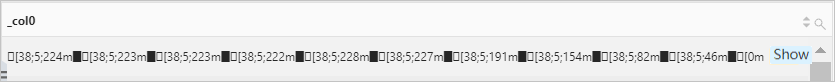• Query and analysis results (server)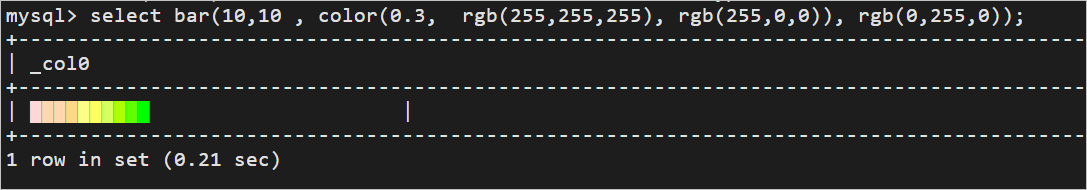## render function

The render function returns results by using color rendering.

### Syntax

• The following function returns results by using color rendering: If the Boolean expression evaluates to true, the function returns a green tick. If the Boolean expression evaluates to false, the function returns a red cross.
``render(boolean expression)``
• The following function returns results by using custom color rendering:
``render(x,color)``

### Parameters

Parameter Description
boolean expression The Boolean expression.
x The X coordinate. The value of this parameter is of the integer type.
color The color. The value of this parameter is of the color type.

### Return value type

The varchar type.

### Examples

• Example 1: Check whether the number of PVs is less than 1,000. The count function returns the number of PVs, and the render function determines whether the number of PVs is less than 1,000 and returns results by using color rendering. If the number of PVs is less than 1,000, the render function returns a green tick.
• Query statement
``* | SELECT render(count(*)<1000)``
• Query and analysis results (console)• Query and analysis results (server)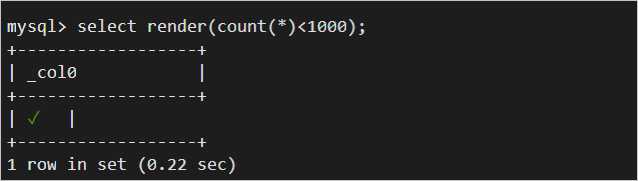• Example 2: Obtain the total number of logs by using green rendering. The count function returns the total number of logs, and the render function returns results by using green rendering.
• Query statement
``* | SELECT render(count(*),rgb(48,169,16))``
• Query and analysis results (console)• Query and analysis results (server)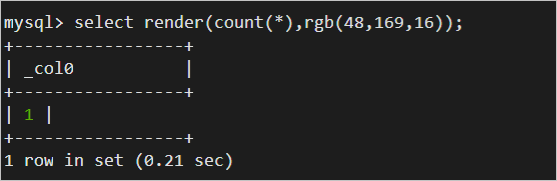## rgb function

The rgb function returns a color value based on an RGB value.

### Syntax

``rgb(red,green,blue)``

### Parameters

Parameter Description
red The portion of red. Valid values: [0,255]. The value of this parameter is of the integer type.
green The portion of green. Valid values: [0,255]. The value of this parameter is of the integer type.
blue The portion of blue. Valid values: [0,255]. The value of this parameter is of the integer type.

The color type.

### Examples

Obtain a color value based on an RGB value.

• Query statement
``*|SELECT rgb(255,0,0)``
• Query and analysis results (console)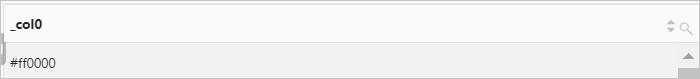• Query and analysis results (server)